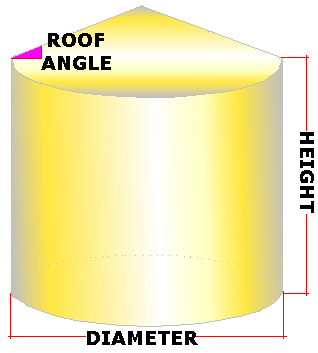Spike's Calculators

# Round Grain Bin Capacity US Bushels

The bushel is a volume measurement and a unit of weight used with various commodities. The United States uses the Winchester bushel, which has a volume equal to 1.244456 cubic feet. In comparison, Canada uses the Imperial ( Avery) bushel, which has a slightly larger volume of 1.284349 cubic feet.

The weight of a bushel is a measure of the grain's density, usually expressed in pounds per bushel (lb/bu) and kilograms per hectolitre (kg/hL).### Formulas

###### The volume of a cylinder;
`π(D/2)²H`
###### Volume of a cone;
`π(D/2)²H/3`
```where π = 3.141593
D = diameter
H = height```
###### Conversions
```one bushel (bu) US = 1.245 cubic feet (ft³)
one cubic foot (ft³) = 0.8036 bushels (bu) US
one pound (lb) = 0.0004114 short tons (tn short)
one pound (lb ) = 0.00045359237 metric tonnes (t)```

### Example Calculation

```Bin height 30 feet
diameter is 40 feet
roof slope is 0 degrees (level)
the grain type is wheat @ 60 lbs per bushel```

`Calculate the volume of the bin;`
```3.141593 * (40 ÷ 2) ² * 30
3.141593 * 400 * 30 = 37699```
`This grain bin has a volume of 37699 cubic feet.`

`Calculate the capacity by multiplying the cubic feet by 0.8036;`
`37699 * 0.8036 = 30295 (rounded up)`
`This bin can hold 30295 US bushels. (Winchester measure)`

`Multiply the number of bushels by the bushel weight of 60 lbs`
`30295 * 60 = 1817695 (rounded up)`
`This bin can hold 1817695 pounds of wheat.`

`Convert the pounds to metric tonnes by multiplying by 0.00045359237;`
`1817695 * 0.00045359237 =  824.49258298715`
`This bin can hold 824.49 metric tonnes of wheat.`

### Grain Bin; Round US Bushels

Diameter ft
Bin Height ft
Slope of Roof °
Bushel Weight lbs

#### Results:

###### Capacity
Peak Height ft
Cubic Feet ft³
US Bushels bu US
Weight lbs
Metric Tons tonnes (t)

#### Calculator

1. enter the diameter of the bin in feet
2. the height of the bin
3. the slope of the roof preset @ 30° (for level set @ 0°)
4. the weight of one bushel

#### Results

1. the height of the peak in feet
2. the volume of the bin in cubic feet
3. the capacity of the bin in US bushels
4. grain weight in pounds
5. the grain weight in metric tonnes